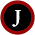Saturday, December 31, 2011

Blob Detection, Connected Component (Pure Opencv)

Connected-component labeling (alternatively connected-component analysis, blob extraction, region labeling, blob discovery, or region extraction) is an algorithmic application of graph theory, where subsets of connected components are uniquely labeled based on a given heuristic. Connected-component labeling is not to be confused with segmentation.

i got the initial code from this URL: http://nghiaho.com/?p=1102
However the code did not compile with my setup of OpenCV 2.2, im guessing it was an older version. so a refactored and corrected the errors to come up with this Class

class atsBlobFinder
{
public:
atsBlobFinder()
{
}
///Original Code by http://nghiaho.com/?p=1102
///Changed and added commments. Removed Errors
///works with VS2010 and OpenCV 2.2+
void FindBlobs(const cv::Mat &binary, vector < vector<cv::Point>  > &blobs)
{
blobs.clear();

// Fill the label_image with the blobs
// 0  - background
// 1  - unlabelled foreground
// 2+ - labelled foreground

///input is a binary image therefore values are either 0 or 1
///out objective is to find a set of 1's that are together and assign 2 to it
///then look for other 1's, and assign 3 to it....so on a soforth

cv::Mat label_image;
binary.convertTo(label_image, CV_32FC1); // weird it doesn't support CV_32S! Because the CV::SCALAR is a double value in the function floodfill

int label_count = 2; // starts at 2 because 0,1 are used already

//erode to remove noise-------------------------------
Mat element = getStructuringElement( MORPH_RECT,
Size( 2*3 + 1, 2*3+1 ),
Point( 0, 0 ) );
/// Apply the erosion operation
erode( label_image, label_image, element );
//---------------------------------------------------

//just check the Matrix of label_image to make sure we have 0 and 1 only
//cout << label_image << endl;

for(int y=0; y < binary.rows; y++) {
for(int x=0; x < binary.cols; x++) {
float checker = label_image.at<float>(y,x); //need to look for float and not int as the scalar value is of type double
cv::Rect rect;
//cout << "check:" << checker << endl;
if(checker == 1) {
//fill region from a point
cv::floodFill(label_image, cv::Point(x,y), cv::Scalar(label_count), &rect, cv::Scalar(0), cv::Scalar(0), 4);
label_count++;
//cout << label_image << endl <<"by checking: " << label_image.at<float>(y,x) <<endl;
//cout << label_image;

//a vector of all points in a blob
std::vector<cv::Point> blob;

for(int i=rect.y; i < (rect.y+rect.height); i++) {
for(int j=rect.x; j < (rect.x+rect.width); j++) {
float chk = label_image.at<float>(i,j);
//cout << chk << endl;
if(chk == label_count-1) {
blob.push_back(cv::Point(j,i));
}
}
}
//place the points of a single blob in a grouping
//a vector of vector points
blobs.push_back(blob);
}
}
}
cout << label_count <<endl;
imshow("label image",label_image);
}
private:
};

From the code comments, ive answered and tested a few parts which the original author did not discuss.

Running on Visual Studio 2010 and OpenCV 2.2 using the 4 connected neighbors and opencv internal function FloodFill.

1. Get the Binary image. the binary image should have values of 0 and 1 only.
2. go through each pixel and find the value 1, floodfill and replace all 1 with a counter ie. number 2
3. every blob found should now be part of floodfill and all neighbors value changed to an ascending number
4. from each blob, get the x and y coordinate of that blob and save it in a vector. giving you a vector<Point>
5. save each of those Vector<Point> into another Vector which represents all the blobs found
6. Output the value

atsBlobFinder blb;
cv::Mat output = cv::Mat::zeros(img.size(), CV_8UC3); //3 Channels

cv::Mat binary;
vector <vector<cv::Point>> blobs;

blb.FindBlobs(binary, blobs);
for(size_t i=0; i < blobs.size(); i++) {
unsigned char r = 255 * (rand()/(1.0 + RAND_MAX));
unsigned char g = 255 * (rand()/(1.0 + RAND_MAX));
unsigned char b = 255 * (rand()/(1.0 + RAND_MAX));

for(size_t j=0; j < blobs[i].size(); j++) {
int x = blobs[i][j].x;
int y = blobs[i][j].y;

output.at<Vec3b>(y,x) = ima.at<uchar>(y,x); //channel 1
output.at<Vec3b>(y,x) = ima.at<uchar>(y,x); //channel 2
output.at<Vec3b>(y,x) = ima.at<uchar>(y,x); //channel 3
}
}
imshow("con comp",output);

the vector<vector<Point>> blobs can create a mask and output the blob in separate images

1.I'm trying to find the centroid of the blobs using this code. I tried something like:

cv::Moments m=cv::moments(blobs);
float xCentroid = m.m10/m.m00;
float yCentroid = m.m01/m.m00;

but this gave me inconsistent results, so I suppose it's wrong. Can you help me correcting it so I can find the centroid of the blobs?

1.hello rafaelperrone,
to find the centroid of the blob, get the min , max value of the blob and divide it by 2. simple.